SSC  >  SSC CPO Mock Test (Quant Apt) 3

# SSC CPO Mock Test (Quant Apt) 3

Test Description

## 50 Questions MCQ Test SSC CPO & Constable - Mock Tests & Previous Year Papers | SSC CPO Mock Test (Quant Apt) 3

SSC CPO Mock Test (Quant Apt) 3 for SSC 2023 is part of SSC CPO & Constable - Mock Tests & Previous Year Papers preparation. The SSC CPO Mock Test (Quant Apt) 3 questions and answers have been prepared according to the SSC exam syllabus.The SSC CPO Mock Test (Quant Apt) 3 MCQs are made for SSC 2023 Exam. Find important definitions, questions, notes, meanings, examples, exercises, MCQs and online tests for SSC CPO Mock Test (Quant Apt) 3 below.
Solutions of SSC CPO Mock Test (Quant Apt) 3 questions in English are available as part of our SSC CPO & Constable - Mock Tests & Previous Year Papers for SSC & SSC CPO Mock Test (Quant Apt) 3 solutions in Hindi for SSC CPO & Constable - Mock Tests & Previous Year Papers course. Download more important topics, notes, lectures and mock test series for SSC Exam by signing up for free. Attempt SSC CPO Mock Test (Quant Apt) 3 | 50 questions in 30 minutes | Mock test for SSC preparation | Free important questions MCQ to study SSC CPO & Constable - Mock Tests & Previous Year Papers for SSC Exam | Download free PDF with solutions
 1 Crore+ students have signed up on EduRev. Have you?
SSC CPO Mock Test (Quant Apt) 3 - Question 1

### Harsha is 40 yrs old and Rith is 60 yrs old. How many yrs ago was the ratio of their ages 3:5?

SSC CPO Mock Test (Quant Apt) 3 - Question 2

### Which one of the following is the solution of the system of linear equations (5/x)-(4/y)=3 and (9/x)-(8/y)=7?

SSC CPO Mock Test (Quant Apt) 3 - Question 3

### Factors of(2x-3y)³+(3y-5z)³+(5z-2x)³ are

SSC CPO Mock Test (Quant Apt) 3 - Question 4
If 2x+3y=26; 2y+z=19 and x+2z=29,what is the value of of x+y+z?
Detailed Solution for SSC CPO Mock Test (Quant Apt) 3 - Question 4 2x + 3y = 26 .....(i)
2y + z = 19 .....(ii)
x + 2z = 29 .....(iii)
from (ii) and (iii)
x + 2(19 - 2y) = 29
x + 38 - 4y = 29
x - 4y = - 9 .....(iv)
from (i), (ii) and (iv) we get
y = 4, x = 7, z = 11
∴ x + y + z = 4 + 7 + 11 = 22
SSC CPO Mock Test (Quant Apt) 3 - Question 5

If α, β, γ and Δ are the roots of the polynominal equation (x2 - 3x + 4)(x2 + 2x + 5)= 0, then the quardratic equation whose roots are α + β + γ + Δ and αβγΔ is

Detailed Solution for SSC CPO Mock Test (Quant Apt) 3 - Question 5

α + β = 3, α.β = 4
γ + Δ = -2, γ.Δ = 5
α + β + γ + Δ = 3 - 2 = 1
α.β.γ.Δ = 4 × 5 = 20
x2 - (α + β + γ + Δ)x + (αβγΔ) = 0
x2 - x + 20 = 0

SSC CPO Mock Test (Quant Apt) 3 - Question 6

Diameter of two circular plates are respectively 18cm and 80 cm. The diameter of the plate which has an area equal to the combined area of two given plates is

Detailed Solution for SSC CPO Mock Test (Quant Apt) 3 - Question 6

Combined area of two plates = π (9)2 + π (40)2
= π (81 + 1600)
= 1681 π cm2.
Let R be the Radius of plate, whose area is combined area of two plates.
Area of plate = πR2 = 1681 π
⇒ R2 = 1681
⇒ R = 41 cm.
Diameter = 2 x 41 = 82 cm

SSC CPO Mock Test (Quant Apt) 3 - Question 7

The average of runs of a cricket player of 10 innings was 32. How many runs must he make in his next innings so as to increase his average of runs by 4?

Detailed Solution for SSC CPO Mock Test (Quant Apt) 3 - Question 7

Let the cricket player makes x runs in his next innings
∴ 32 × 10 + x = 11 × 36
∴ x = 396 - 320 = 76

SSC CPO Mock Test (Quant Apt) 3 - Question 8

OABC is a rhombus whose vertices A, B and C lie on a circle with centre at O. If radius of circle is 10cm. The area of the rhombus

Detailed Solution for SSC CPO Mock Test (Quant Apt) 3 - Question 8

If A, B, and C lie on the circle, O is the center of the circle, and the radius is 10 cm, then OA, OB, OC, AB, and BC must all measure 10 cm because OA, OB, and OC are all radii, and AB and BC are congruent because of congruent sides of a rhombus.
Hence, the area of the rhombus is twice the area of an equilateral triangle with sides that measure 10 cm.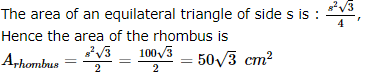SSC CPO Mock Test (Quant Apt) 3 - Question 9

Diagonals of a rhombus are in the ratio 3:4 and its area is 2400 cm2. Side of the rhombus is

Detailed Solution for SSC CPO Mock Test (Quant Apt) 3 - Question 9

Let the diagonals of a rhombus are 3K and 4K. Then
Area of rhombus = 1/2 x product of diagonals
2400 = 1/2 x 3K x 4K
or 400 = K2
or K = 200
∴ The Diagonals are 60 cm and 80 cm
we know that, if a be side of the rhombus and AC and BD be its diagonals then
(AC)2 + (BD)2 = 4 × a2
or (60)2 + (80)2 = 4 × a2
or 3600 + 6400 = 4 × a2
or 10000 = 4a2
or 2500 = a2
or a = 50 cm

SSC CPO Mock Test (Quant Apt) 3 - Question 10
The average of 5 numbers is 27. If one number is excluded,the average becomes 25. The excluded number is
SSC CPO Mock Test (Quant Apt) 3 - Question 11

The equation x² + Kxy + y² - 5x - 7y + 6 = 0 represents a pair of st. lines, then K is

SSC CPO Mock Test (Quant Apt) 3 - Question 12

Find Compound Interest on Rs 15625 for 9 months at 16% p.a compounded quarterly.

Detailed Solution for SSC CPO Mock Test (Quant Apt) 3 - Question 12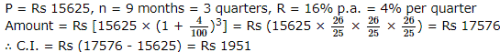SSC CPO Mock Test (Quant Apt) 3 - Question 13

A shopkeeper marks his goods 20% above the cost price but allows 30% discount for cash. His net loss is

Detailed Solution for SSC CPO Mock Test (Quant Apt) 3 - Question 13

Let C.P. of goods = Rs. 100
∴ Marked price = 100 + 20
= Rs 120
∴ Selling price after allowing 30% discount for cash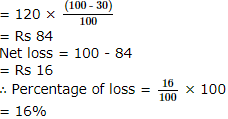SSC CPO Mock Test (Quant Apt) 3 - Question 14

Which one of the following is the least?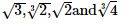SSC CPO Mock Test (Quant Apt) 3 - Question 15

Simplify : [(0.2×0.2+0.2×0.02)/(0.044)]

SSC CPO Mock Test (Quant Apt) 3 - Question 16
In ∆ABC, ∠B = 90�, AB = 6 cm and BC = 8 cm. Its circum radius is
Detailed Solution for SSC CPO Mock Test (Quant Apt) 3 - Question 16 Using Pythogorous theorem AC = 10 cm. Circum radius of a right triangle is half the hypotenuse. Hence r = 5 cm.
SSC CPO Mock Test (Quant Apt) 3 - Question 17

If the magnitude of angle θ is three times that of the angle φ in the given figure, then ∠φ is equal to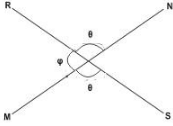SSC CPO Mock Test (Quant Apt) 3 - Question 18
If AB and CD are two parallel straight lines and EF is a transversal which cuts AB at P and CD at Q, then the angles APQ and PQD are called :
SSC CPO Mock Test (Quant Apt) 3 - Question 19
Lines l and m are parallel. If p and q are two distinct lines such that p $\perp$ l and q $\perp$ m, then
Detailed Solution for SSC CPO Mock Test (Quant Apt) 3 - Question 19 If two distinct lines are perpendicular to two parallel lines then they are parallel to each other.
SSC CPO Mock Test (Quant Apt) 3 - Question 20
The least number, which when divided by 4,5 and 6 leaves the remainder 1,2 and 3 respectively, is
SSC CPO Mock Test (Quant Apt) 3 - Question 21
How much is the greatest number of three digits less than the smallest number of three digits?
SSC CPO Mock Test (Quant Apt) 3 - Question 22

The angle of elevation of the top of  an incomplete tower at a point 40 m from its base is 45°,If the elevation of the completed tower at the same point is 60°,then the height through which the tower has been raised is

SSC CPO Mock Test (Quant Apt) 3 - Question 23
When the price of a product was increased by 15% the number sold was decreased by 20%. What was the net effect?
SSC CPO Mock Test (Quant Apt) 3 - Question 24
Rajeev buys goods worth Rs.6650. He gets a rebate of 6% on it. After getting the rebate, he pays sales tax @10%. Find the amount he will have to pay for the goods.
SSC CPO Mock Test (Quant Apt) 3 - Question 25

2 pipes A and B can fill a cistern in 20 minutes and 25 minutes respectively, Both are opened together but at the end of 5 minutes B is turned off. Total time taken to fill the cisten is

Detailed Solution for SSC CPO Mock Test (Quant Apt) 3 - Question 25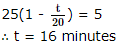SSC CPO Mock Test (Quant Apt) 3 - Question 26
A merchant bought 10 items for Rs 200 each. He sold 5 of them at a profit of 10%. The trader wants to have on overall profit of 15%. The price at which he should sell each of the remaining items is
SSC CPO Mock Test (Quant Apt) 3 - Question 27
A man sells two articles at Rs 99 each. On one he gains 10% and on other he loses 10%. What is his gain or loss percent on the whole transaction?
SSC CPO Mock Test (Quant Apt) 3 - Question 28

In ΔABC , if a , b , c , are in A.P . , then tan A/2 tan C/2 =

SSC CPO Mock Test (Quant Apt) 3 - Question 29
A sum of money is to be divided among P,Q and R in the ratio of 3:7:13 respectively. If the total share of P and Q together is Rs 20,000, what is the difference between the shares of Q and R?
SSC CPO Mock Test (Quant Apt) 3 - Question 30
Two numbers are in the ratio of 5:7. On diminishing each of them by 40, they become in the ratio 17:27. The difference of the numbers is
SSC CPO Mock Test (Quant Apt) 3 - Question 31
Let the harmonic means and the geometric mean of two positive numbers be in the ratio 4 : 5. The two numbers are in the ratio
SSC CPO Mock Test (Quant Apt) 3 - Question 32
A money lender charged Rs 120 as simple interest on a sum of Rs 400 for four months. The rate of interest per annum will be
SSC CPO Mock Test (Quant Apt) 3 - Question 33
The square root of 0.9 is equal to
SSC CPO Mock Test (Quant Apt) 3 - Question 34
Points A and B are 60 km apart. One bus starts from A and another bus starts from B at the same time. If they go in the same direction, they meet after 6 hrs and if they go in opposite directions they meet after 2 hrs. The speed of the bus with the greater speed is
SSC CPO Mock Test (Quant Apt) 3 - Question 35
Rama can complete a job in 8 days. Shyamu can complete the same job in 12 days. However, Monu takes 12 days to complete 3/4th of the same job. Ramu works for 2 days and then leaves a job. The number of days will be taken by Shyamu and Monu to complete the balance together is
SSC CPO Mock Test (Quant Apt) 3 - Question 36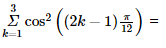SSC CPO Mock Test (Quant Apt) 3 - Question 37

If x sin³α+y cos³α=sinα cosα and x sinα-y cosα=0, then x²+y²=

SSC CPO Mock Test (Quant Apt) 3 - Question 38

A solid cylindrical block of radius 12 cm and height 18 cm is mounted with a conical block of radius 12 cm and height 5 cm. The total lateral surface of the solid thus formed is :

Detailed Solution for SSC CPO Mock Test (Quant Apt) 3 - Question 38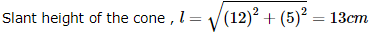Lateral surface of the solid = Curved surface of cone + Curved surface of cylinder + Surface area of bottom
= πrl + 2πrh + πr2, where h is the height of the cylinder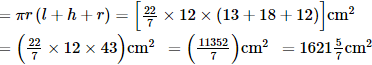SSC CPO Mock Test (Quant Apt) 3 - Question 39
1+cos2x+cos4x+cos6x=
SSC CPO Mock Test (Quant Apt) 3 - Question 40

The edges of a cuboid are in the ratio 1:2:3 and its surface area is 88 cm². The volume of the cuboid is :

SSC CPO Mock Test (Quant Apt) 3 - Question 41
The volumes of two spheres are in the ratio of 64:27. The ratio of their surface areas is :
SSC CPO Mock Test (Quant Apt) 3 - Question 42

Study the graph given below to answer these questions.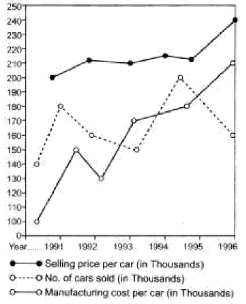Q. In which year, were the gross sales proceeds the highest?

Detailed Solution for SSC CPO Mock Test (Quant Apt) 3 - Question 42

Sales proceeds in the year
1992 = 180 × 210 = Rs 37800 million
Sales proceed in the year
1993 = 160 × 207 = Rs 33120 million
Sales proceeds in the year
1994 = 150 × 210 = Rs 31500 million
Sales in the year
1995 = 195 × 210 = Rs 40950 million
It is highest in the year 1995

SSC CPO Mock Test (Quant Apt) 3 - Question 43

Study the graph given below to answer these questions.Q. Which year was the least profitable from the fiscal point of veiw ?

Detailed Solution for SSC CPO Mock Test (Quant Apt) 3 - Question 43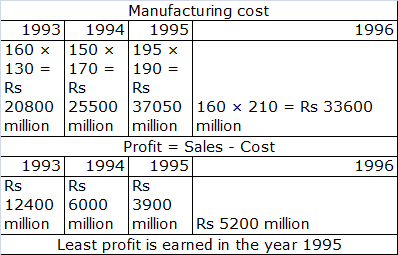SSC CPO Mock Test (Quant Apt) 3 - Question 44

Study the graph given below to answer these questions.Q. In which year the number of cars sold was the lowest but financial turnover , the maximum ?

Detailed Solution for SSC CPO Mock Test (Quant Apt) 3 - Question 44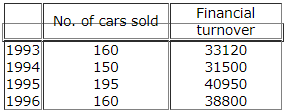The year 1996 the number of cars sold is least and financial turnover maximum

SSC CPO Mock Test (Quant Apt) 3 - Question 45

Study the graph given below to answer these questions.Q. In which year, the maximum profit was generated vis-a-vis in ?

Detailed Solution for SSC CPO Mock Test (Quant Apt) 3 - Question 45

In the 1991 profit generated is the maximum

SSC CPO Mock Test (Quant Apt) 3 - Question 46

These questions refer to the following circle graph showing the expenditure distribution of a certain family.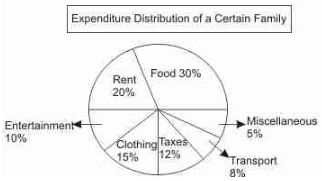If the family spends Rs.6,500 per month, how much are its annual taxes ?

Detailed Solution for SSC CPO Mock Test (Quant Apt) 3 - Question 46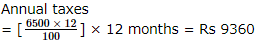SSC CPO Mock Test (Quant Apt) 3 - Question 47

These questions refer to the following circle graph showing the expenditure distribution of a certain family.Q. How many degrees should there be in the central angle showing clothing , taxes and transportation combined ?

Detailed Solution for SSC CPO Mock Test (Quant Apt) 3 - Question 47

Required angle
= 360/100 × 35 = 126°

SSC CPO Mock Test (Quant Apt) 3 - Question 48

These questions refer to the following circle graph showing the expenditure distribution of a certain family.Q. How much more money per month is spent by the family on food as compared to the rent ?

Detailed Solution for SSC CPO Mock Test (Quant Apt) 3 - Question 48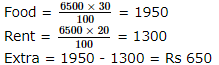SSC CPO Mock Test (Quant Apt) 3 - Question 49

These questions refer to the following circle graph showing the expenditure distribution of a certain family.Q. If the expenditure budget of the family is raised to Rs.8,000 per month and distribution on various items remain the same , then the monthly expenses on both , the entertainment and the transport, will be

Detailed Solution for SSC CPO Mock Test (Quant Apt) 3 - Question 49

Expenses on entertainment and transport
= 8000 × 18%
= Rs 1440

SSC CPO Mock Test (Quant Apt) 3 - Question 50

The pie chart given below shows the funding arrangements for National Highways Development Projects: phase I. Study the chart carefully to answer these questions.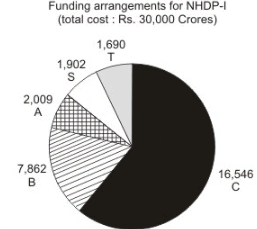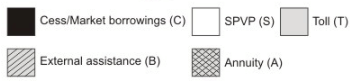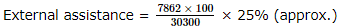Q. Near about 25% of the funding arrangement is through :

Detailed Solution for SSC CPO Mock Test (Quant Apt) 3 - Question 50## SSC CPO & Constable - Mock Tests & Previous Year Papers

1 docs|63 tests
 Use Code STAYHOME200 and get INR 200 additional OFF Use Coupon Code
Information about SSC CPO Mock Test (Quant Apt) 3 Page
In this test you can find the Exam questions for SSC CPO Mock Test (Quant Apt) 3 solved & explained in the simplest way possible. Besides giving Questions and answers for SSC CPO Mock Test (Quant Apt) 3, EduRev gives you an ample number of Online tests for practice

1 docs|63 tests